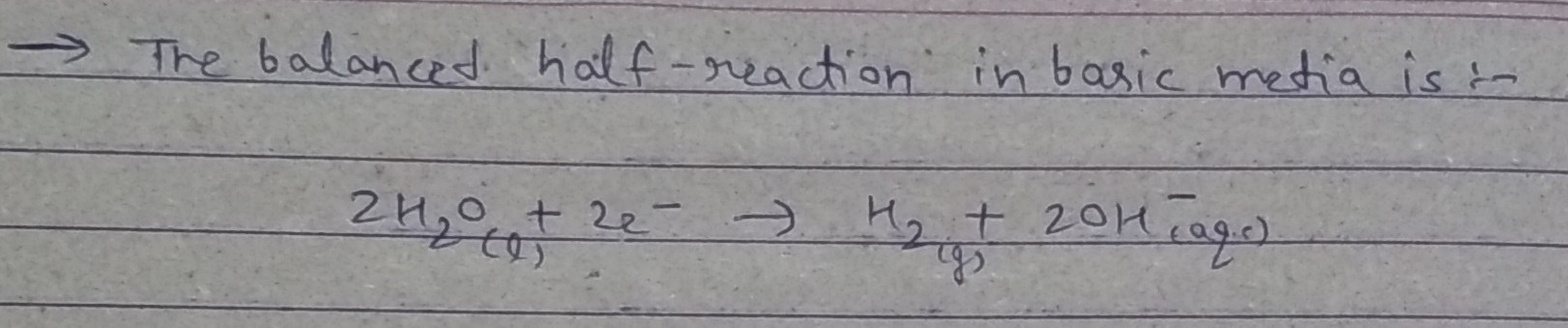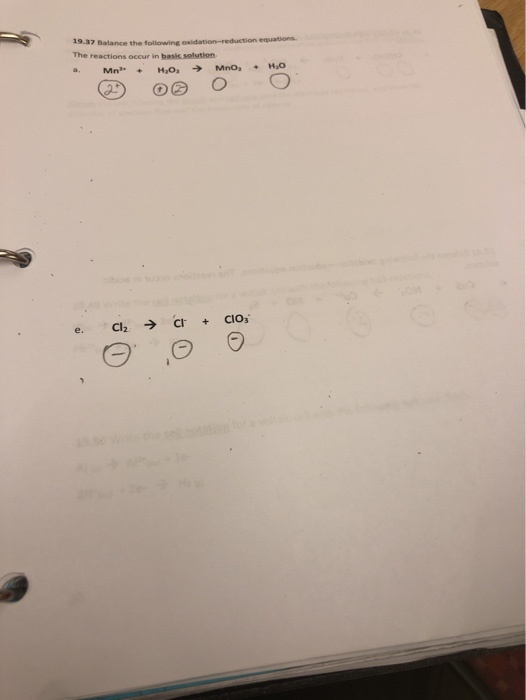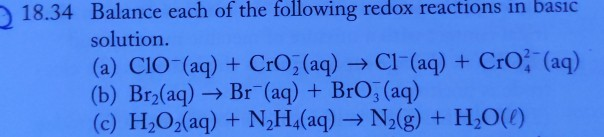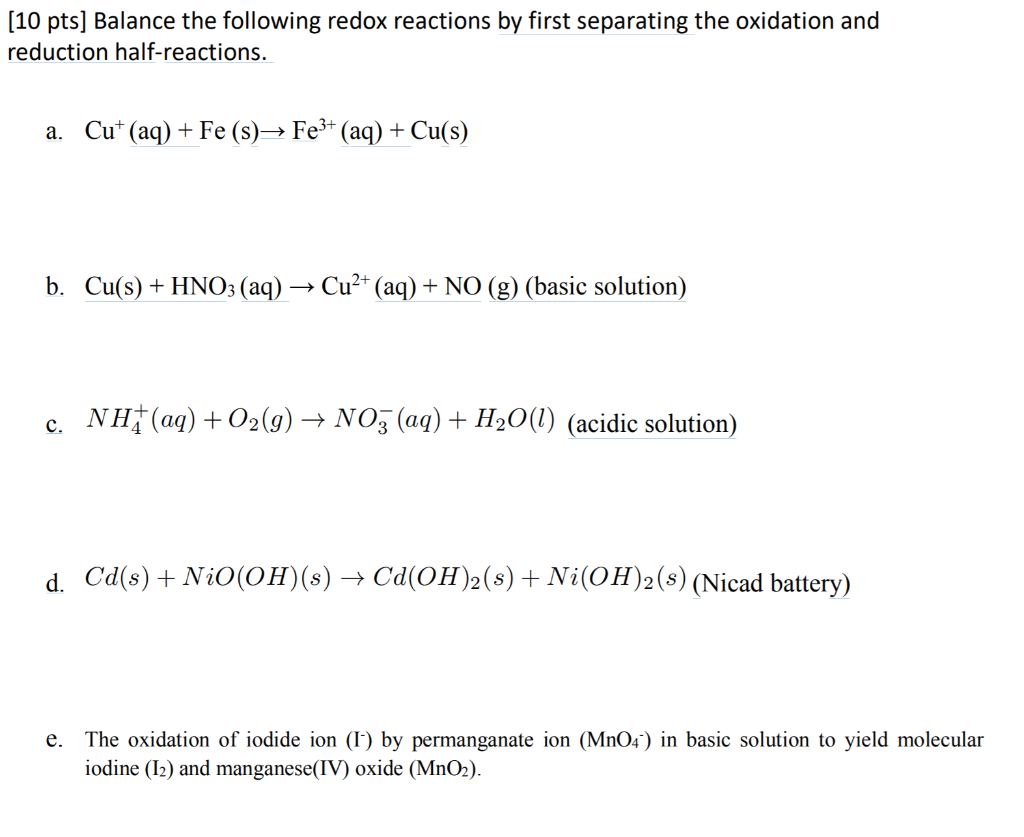Question

# Balance each of the following half-reactions, assuming that they occur in basic solution. H2O(l)→H2(g)

Balance each of the following half-reactions, assuming that they occur in basic solution.

H2O(l)→H2(g)#### Earn Coins

Coins can be redeemed for fabulous gifts.

Similar Homework Help Questions
• ### 19.37 Balance the following oxidation-reduction equations The reactions occur in basic solution a. Mn + H2O...19.37 Balance the following oxidation-reduction equations The reactions occur in basic solution a. Mn + H2O Mno, - HO e. Cl2 + C + Cio;

• ### Balance each of the following redox reactions in basic solution. 18.34 Balance each of the following...Balance each of the following redox reactions in basic solution. 18.34 Balance each of the following redox reactions in basic solution. (a) CIO-(aq) + CrO(aq) → C1- (aq) + Cro (aq) (b) Br2(aq) + Br (aq) + BrO3(aq) (c) H2O2(aq) + N H(aq) → N2(g) + H2O(O)

• ### You are given the following reduction half reactions in basic solution: 2 NO2-(aq) + 3 H2O(l)...

You are given the following reduction half reactions in basic solution: 2 NO2-(aq) + 3 H2O(l) + 4 e- →N2O(g) + 6 OH-(aq) E° = 0.1500 V HgO(s) + H2O(l) + 2 e- → Hg(s) + 2 OH-(aq) E° = +0.0984 V A. If this redox reaction occurs, how many total electrons will have to be transferred per mole of reaction? N2O(g) + 2 OH-(aq) + 2 HgO(s) → 2 NO2-(aq) + H2O(l) + 2 Hg(s) B. What is the...

• ### Complete and balance the following half-reactions. In each case indicate whether the...

Complete and balance the following half-reactions. In each case indicate whetherthe half-reaction is an oxidation or a reduction.N_2(g) --> NH_4^+(acidic solution)SO_3^2-(aq) --> SO_4^2-(aq) (basic solution)N2(g) --> NH_3(g) (basic solution)

• ### Find the Half-Reactions of the following equation in a BASIC solution, Balance the basic equation, and...

Find the Half-Reactions of the following equation in a BASIC solution, Balance the basic equation, and indicate which substances are oxidizing and reducing agents: CrI3(s) + H2O2(aq) = CrO4^2-(aq) + IO4-(aq)

• ### Balance the following half-reaction CH3CHO(l) → CO2(g) for basic solution using the smallest possible integer coefficients.

Balance the following half-reaction CH3CHO(l) → CO2(g) for basic solution using the smallest possible integer coefficients.

• ### Classify the half‑reactions as reduction half‑reactions or oxidation half‑reactions. H2(g)⟶2H+(aq)+2e−H2(g)⟶2H+(aq)+2e− 12O2(g)+2H+(aq)+2e−⟶H2O(g)12O2(g)+2H+(aq)+2e−⟶H2O(g) Cd(s)+2OH−(aq)⟶Cd(OH)2(s)+2e−Cd(s)+2OH−(aq)⟶Cd(OH)2(s)+2e− 2NiO(OH)(s)+2H2O(l)+2e

Classify the half‑reactions as reduction half‑reactions or oxidation half‑reactions. H2(g)⟶2H+(aq)+2e−H2(g)⟶2H+(aq)+2e− 12O2(g)+2H+(aq)+2e−⟶H2O(g)12O2(g)+2H+(aq)+2e−⟶H2O(g) Cd(s)+2OH−(aq)⟶Cd(OH)2(s)+2e−Cd(s)+2OH−(aq)⟶Cd(OH)2(s)+2e− 2NiO(OH)(s)+2H2O(l)+2e−⟶2Ni(OH)2(s)+2OH−(aq)2NiO(OH)(s)+2H2O(l)+2e−⟶2Ni(OH)2(s)+2OH−(aq) Fe(s)⟶Fe2+(aq)+2e−Fe(s)⟶Fe2+(aq)+2e− oxidation reduction reduction oxidation reduction

• ### (10 pts) Balance the following redox reactions by first separating the oxidation and reduction half-reactions. a....(10 pts) Balance the following redox reactions by first separating the oxidation and reduction half-reactions. a. Cut (aq) + Fe (s) Fe3+ (aq) + Cu(s) b. Cu(s) + HNO3 (aq) Cu2+ (aq) + NO (g) (basic solution) c. NH(aq) + O2(g) → N03 (aq) + H2O(l) (acidic solution) d. Cd(s) + NiO(OH)(s) + Ca(OH)2(s) + Ni(OH)2(s) (Nicad battery) e. The oxidation of iodide ion (1) by permanganate ion (MnO4) in basic solution to yield molecular iodine (12) and manganese(IV) oxide...

• ### Balance the following equation by the half-reaction method. The reaction takes place in basic solution. Fe(s) + H2O(1)...Balance the following equation by the half-reaction method. The reaction takes place in basic solution. Fe(s) + H2O(1) + O2(g) → Fe(OH)3(s) Express your answer as a chemical equation including phases.

• ### balance the following half-reactions (all of which take place in acidic solution) a. HClO(aq) ---> CL^-(AQ)...

balance the following half-reactions (all of which take place in acidic solution) a. HClO(aq) ---> CL^-(AQ) b. NO(AQ)--->N2O(G) c. N2O(AQ)--->N2(G) d. CLO3^-(AQ--->HCLO2(AQ) e. O2(G)--->H2O(L) f. SO4^2^-(AQ)--->H2SO3(AQ) g. H2O2(AQ)--->H2O(L) h. NO2^-(AQ)--->NO3^-(AQ)